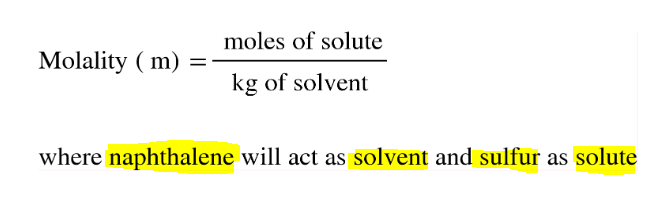# Problem: How many grams of sulfur (S8) must be dissolved in 100.0 g naphthalene (C10H8) to make a 0.13 m solution?

###### FREE Expert Solution
• Molality can be calculated as:• We will be solving for the moles of Sfirst and then mass of S8 using its molar mass

• Molar mass of S8 is:

S - 32.07 (8) = 256.56 g/mol

100% (418 ratings)###### Problem Details

How many grams of sulfur (S8) must be dissolved in 100.0 g naphthalene (C10H8) to make a 0.13 m solution?